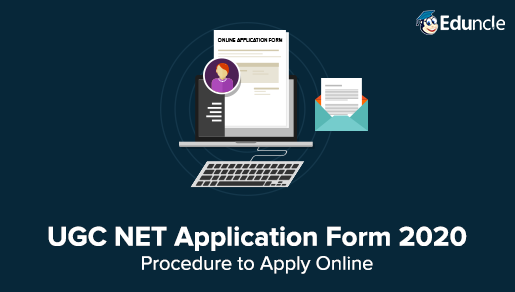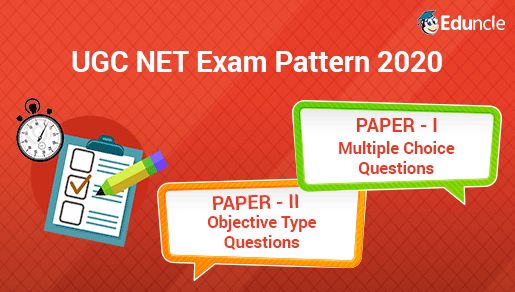UGC NET FollowSaloni Jain @Eduncle posted an Article
December 18, 2019 • 12:08 pm

# How to Solve UGC NET Paper 1 Data Interpretation Questions in Minutes?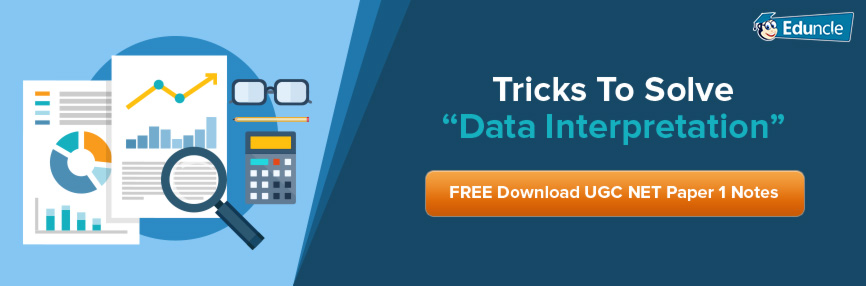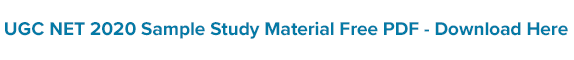Data Interpretation is one of the most important topics for UGC NET Paper 1 Exam. It holds around 10-12 marks in the exam with the occurrence of 5-6 questions from this single section.

The students who think that they can easily crack this section without putting much efforts are looming around the myth.

However, the Data Interpretation questions that come in UGC NET are not as tough as in the Government Competitive Exams, but their difficulty level remains high.

As seen in the previous years' exams, the Data-Interpretation Section in UGC NET Paper 1 contains mostly questions from Table and PI Chart topics. Any student who studies this section for 15-20 days can master its formulas and concepts thoroughly to score enough marks in the NTA NET Exam.

Here in this blog, Eduncle Mentors have shared some of the Best Techniques & Formulas to solve UGC NET Data Interpretation Questions with ease.

## Tricks to Crack Data Interpretation Section in UGC NET with 10/10 Score

UGC NET Data Interpretation questions would never be as easier as you think. From moderate to from high difficulty level, the questions picked from Data Interpretation section would always be tricky to solve.

To study smart and win over others, you need to filter out which topics are more important, and which are less.

Must Read – How to Study for UGC NET Exam June 2020?

### Important Topics under UGC NET General Aptitude Data Interpretation Section

As per the UGC NET Syllabus for paper 1, there are around 10-12 major topics for exam perspective. Here in this blog, we’re presenting the learning tricks and tips of DI section, therefore the important topics for the same section are given below -

Sources, acquisition, and interpretation of data

Quantitative and qualitative data

Graphical representation and mapping of data

Bar Chart

Gantt Chart

Line Chart

Pie Chart

Polygraphs

Maps

Pictograms

Flow Chart and Organization Chart

Out of the above topics, the students can start study/practice DI questions in the following order –

Table based DI

Pie Chart

Line Graph

Bar Chart

Download the Free Copy of UGC NET June 2020 Syllabus & Start Up Your Preparation Journey Now Onwards.

### UGC NET Paper 1 Data Interpretation - Daily Time Management Plan for 15 Days of Learning

Spend 2 hours daily till 15 days and you’re done with the UGC NET Paper 1 Data Interpretation Topic.

Try to save morning and evening hours for better practicing.

Wait! before proceeding ahead, download NTA NET Sample Papers and Previous Years Papers to have an ample number of questions to practice from.

Download these question papers and start your 15-day challenge to finish up the preparations for UGC NET Paper 1 Data Interpretation (DI) Section.

### Solve Data Interpretation Questions in Minutes with These Experts Recommended Techniques

1. The majority of questions asked in the Data interpretation, Section is based on the following topics of the Arithmetic Section.

(i) Ratios

(ii) Averages

(iii) Percentage

If the basics of these topics are clear, attempting DI in the UGC NET Question Paper becomes comparatively easy.

2. Data Interpretation questions are based on information given in tables and graphs.

These questions test your ability to interpret the information presented and to select the appropriate data for answering a question.

3. Analyze and read the given question as well as its information carefully. This will help you in framing the right answer within minutes.

4. Avoid lengthy calculations! Generally, data interpretation questions do not require extensive calculations and computations.

Most questions simply require reading the data correctly and putting things together to use them directly with common sense.

5. Breakdown lengthy questions into smaller parts and eliminate impossible choices.

6. Use only the information given and your knowledge of everyday facts, such as the number of hours in a day, to answer the questions based on tables and graphs.

7. Stick to the question and its requirements. Don’t go with the assumptions, otherwise, you may lead to the wrong answer and lose your marks.

8. Be careful while dealing with units.

9. To make reading easier and to avoid errors, observe graphs while keeping them straight.

10. Be prepared to apply basic mathematical rules, principles and formulae.

11. Since one of the major benefits of graphs and tables is that they present data in a form that enables you to readily make comparisons. Use this visual attribute of graphs and tables to help you answer the questions. Where possible, use your eyes instead of your computational skills.

12. Practice, Practice, Practice: The last but the most important piece of advice. Practicing questions will help you in two ways:

Your speed will increase considerably. You would be able to attempt all kinds of DI questions many numbers of times. You will end up attempting more questions correctly, thus increasing your score.

Your confidence will increase considerably. You know you have practiced a lot! This will help you face tough questions with determination.

## UGC NET Data Interpretation Paper 1 | Top Formulas to Solve Questions with 100% Accuracy

(i) To calculate p % of y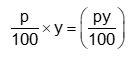p % of y = y % of p

(ii) To find what percentage of x is y =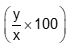(iii) To calculate percentage change in value =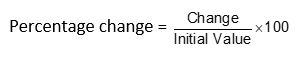(iv) Percentage point change = Difference of two percentage figures

(v) Increase N by S % = N(1+ S/100)

(vi) Decrease N by S % = N (1 – S/100)

(vii) If the value of an item goes up/down by x%, the percentage reduction/increment to be now made to bring it back to the original point is 100x/ (100 + x) %.

(viii) If A is x% more /less than B, then B is 100x/(100 + x) % less/more than A.

(ix) If the price of an item goes up/down by x %, then the quantity consumed should be reduced by [100x / (100+x)]%, so that the total expenditure remains the same.

(x) Successive Percentage Change

If there are successive percentage increases of a % and b%, the effective percentage increase is: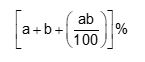(xi) If the price of a commodity increase by P%, then the reduction in consumption so as not to Increase the expenditure is: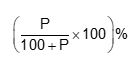(xii) If the price of a commodity decreases by P%. then the increase in consumption so as not to decrease the expenditure is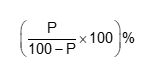## UGC NET Paper 1 Data Interpretation Questions | Solve & Analyze Where You Stand

Eduncle experts have summed up some major questions and have provided their detailed explanation along.

For better practicing, we’d advise you to first solve the questions and then check out their answers.

Let’s start here!

Direction (Q. 1-5): Study the following table to answer the questions that are given below.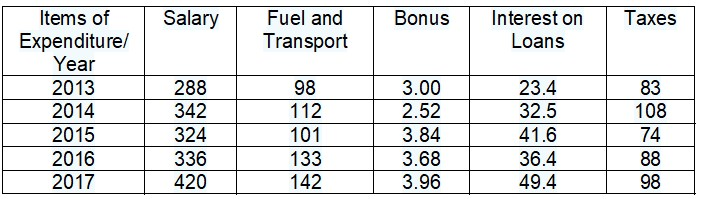1. The ratio between the total expenditure on taxes for all the years and the total expenditure on Fuel and Transport for all the years respectively is approximate:

(A)      4:7                                                     (B)      10:13

(C)      15:18                                                 (D)      5:8

2. The total expenditure of the company on these items during the year 2015 is

(A)      544.44 Lakhs                                               (B)      501.11 Lakhs

(C)      446.46 Lakhs                                               (D)      478.87 Lakhs

3. What is the average amount of interest per year which the company had to pay during this period?

(A)      32.43 Lakhs                                     (B)       33.72 Lakhs

(C)      34.18 Lakhs                                     (D)      36.66 Lakhs

4. Total expenditure on all these items in 2013 was approximately what percent of the total expenditure in 2017?

(A)      62%                                                    (B)       66%

(C)       69%                                                    (D)      71%

5. The total amount of bonus paid by the company during the given period is approximately what percent of the total amount of salary paid during this period?

(A)     0.1%                                                  (B)      0.5%

(C)     1%                                                     (D)     1.25%

Solutions

1. (B)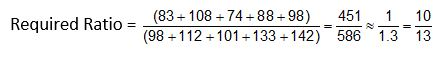2. (A)

Total expenditure of the company during 2015

= Rs. (324 + 101 + 3.84 + 41.6 + 74) lakhs

= Rs. 544.44 lakhs

3. (D)

Average amount of interest paid by the company during the given period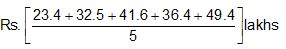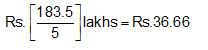4. (C)

Required percentage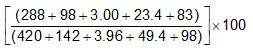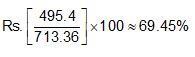5. (C)

Required percentage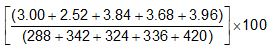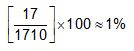Stuck anywhere in solving the DI questions? Register Here for Free to Talk with Eduncle Counsellors & Get Guidance from them!

Also, check out last minute tips for UGC NET Exam to never skip the things which can boost your scores only with the last-minute preparation.

We hope that the Data Interpretation Guide for NTA NET General Paper 1 would surely help you in mastering the formulas, methods and core concepts. We’d like to know your feedback for the same.

Please drop us a line in the comments box and do share your valuable suggestions, queries or feedback for the tips and tricks give above.

All the Best Folks!

You Might Also Like